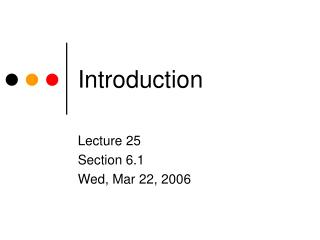DownloadDownload PresentationIntroduction

# Introduction

Download Presentation## Introduction

- - - - - - - - - - - - - - - - - - - - - - - - - - - E N D - - - - - - - - - - - - - - - - - - - - - - - - - - -
##### Presentation Transcript

1. Introduction Lecture 25 Section 6.1 Wed, Mar 22, 2006

2. What is Probability? • A coin has a 50% chance of landing heads. • What does that mean? • The coin will land heads 50% of the time? • This is demonstrably false. • The coin will land heads approximately 50% of the time? • Then the probability is approximately 50%, not exactly 50%.

3. The Meaning of Probability • It means that the fraction of the time that the coin lands heads will get arbitrarily close to 50% as the number of coin tosses increases without bound. • This involves the notion of a limit as n approaches infinity.

4. The Sample Space • An experiment is a procedure that leads to an outcome. • If at least one step in the procedure is left to chance, then the outcome is unpredictable. • We observe a characteristic of the outcome. • The sample space is the set of all possible observations.

5. The Sample Space • Example • Procedure: Toss a coin. • Observed characteristic: Which side landed up. • Sample space = {H, T}

6. The Sample Space • Example • Procedure: Roll a die. • Observed characteristic: Which number landed up. • Sample space = {1, 2, 3, 4, 5, 6}

7. Calculation of Probability • We will consider only finite sample spaces. • If the n members of the sample space are equally likely, then the probability of each member is 1/n. • Examples • Toss a coin, P(H) = 1/2. • Roll a die, P(3) = 1/6.

8. The Probability of an Event • An event is a collection of possible observations, i.e., a subset of the sample space. • The probability of an event is the sum of the probabilities of its individual members. • If the members of the sample space are equally likely, then P(E) = |E|/|S|.

9. Example: Probability of an Event • In a full binary search tree of 25 values, what is the probability that a search will require 5 comparisons? • Assume that all 25 values are equally likely. • 10 of them occupy the bottom row. • Therefore, p = 10/25 = 40%.

10. Example • A deck of cards is shuffled and the top card is drawn. • What is the probability that it is • The ace of spades? • An ace? • A spade? • A black card?

11. Example • A deck of cards is shuffled, the top card is discarded, and the next card is drawn. • What is the probability that it is • The ace of spades? • An ace? • A spade? • A black card?

12. Example • A deck of cards is shuffled, the top card is drawn, and it is noted that it is red. Then the next card is drawn. • What is the probability that it is • The ace of spades? • An ace? • A spade? • A black card?

13. Example • A deck of cards is shuffled, the top card is drawn, and it is noted that it is black. Then the next card is drawn. • What is the probability that it is • The ace of spades? • An ace? • A spade? • A black card?

14. Example • Two red cards and two black cards are laid face down. • Two of them are chosen at random and turned over. • What is the probability that they are the same color?

15. The Monty Hall Problem • See p. 301. • There are three doors on the set for a game show. Call them A, B, and C. • You get to open one door and you win the prize behind the door. • One of the doors has a Ferrari behind it. • You pick door A.

16. The Monty Hall Problem • However, before you open it, Monty Hall opens door B and shows you that there is a goat behind it. • He asks you whether you want to change your choice to door C. • Should you change your choice or should you stay with door A?

17. The Monty Hall Problem • There are three plausible strategies. • Stay with door A. • Door C still has a 1/3 chance, so door A must have a 2/3 chance. • Switch to door C. • Door A still has a 1/3 chance, so door C must have a 2/3 chance. • It doesn’t matter. • Both doors now have a 1/2 chance.

18. The Monty Hall Problem • Use a simulation to determine the correct answer. • MontyHall.exe.

19. A Contest Problem • If we choose an integer at random from 1 to 1000, what is the probability that it can be expressed as the difference of two squares?